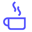a concise shot of dev knowledge
Become a Contributor
Concise shots of dev knowledge

RELATED TAGS

basic
floor
division

# Floor divisionEdpresso Team

Floor division is a normal division operation except that it returns the largest possible integer. This integer is either less than or equal to the normal division result.

Floor function is mathematically denoted by this ⌊ ⌋ symbol.

Let’s understand this concept through the slides below:

1 of 4

## Code

Several programming languages have a specific built-in function or operator for calculating floor division.

• floor() method is used in C++
• Math.floor() is used in Java
• // operator is used in Python
#include <iostream>
//include math library
#include <cmath>

using namespace std;

int main() {
// floor() method is used.
cout << "Floor of 36/5 is " << floor(36/5) << endl;
return 0;
}

RELATED TAGS

basic
floor
division
RELATED COURSES

View all Courses

Keep Exploring

Learn in-demand tech skills in half the time